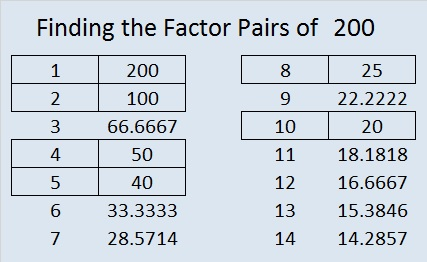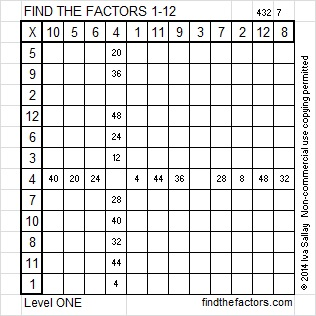# 200 and Level 1

 200 is a composite number. Factor pairs: 200 = 1 x 200, 2 x 100, 4 x 50, 5 x 40, 8 x 25, or 10 x 20 Factors of 200: 1, 2, 4, 5, 8, 10, 20, 25, 40, 50, 100, 200 Prime factorization: 200 = 2 x 2 x 2 x 5 x 5, which can be written 200 = (2^3) x (5^2)200 is the smallest positive number that cannot be turned into a prime number by changing ONE of its digits to a different digit. If you can remember that little fact, if should help you to remember that ALL the numbers from 200 to 210 are composite numbers.Excel file of puzzles and previous week’s factor solutions: 12 Factors 2014-08-111.wbhs62